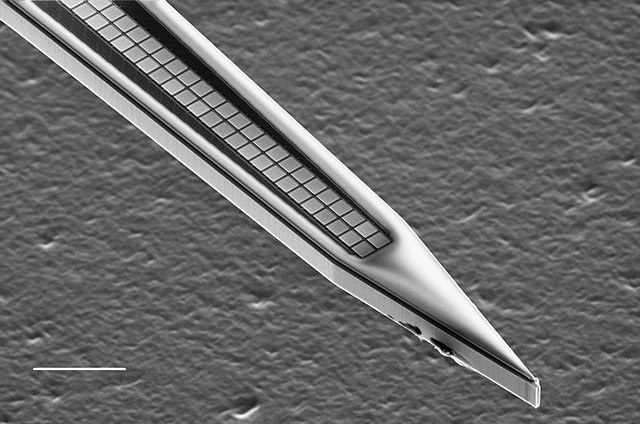Justin Kiggins

Product. Data. Science.

# Defining Large Array Geometries for Klustakwik

02 July 2015

We’ve started using KlustaKwik for spike sorting in the lab and I’ve been working on getting a data pipeline going. It’s masked EM algorithm is very clever, taking into account the adjacency matrix of an electrode array to determine whether it should use one, two, or n recording sites when clustering.

However, very large arrays make it unrealistic to manually define the probe architectures that SpikeDetekt2 expects. For example, the dense silicon arrays designed by Ed Boyden’s group:5 shanks with 200 sites each and just 11 microns pitch between sites

This example programmatically builds a `channel_groups` dictionary for a large array, using just a handful of parameters of the array.

### first, we need to be able to convert a geometry into a graph

we’ll define a function which takes the `geometry` dict, extracts the coordinates, and uses the Delaunary transformation to generate a triangular tesselation. It then returns this graph in the form that SpikeDetekt2 expects

``````from scipy import spatial
from scipy.spatial.qhull import QhullError
def get_graph_from_geometry(geometry):

# let's transform the geometry into lists of channel names and coordinates
chans,coords = zip(*[(ch,xy) for ch,xy in geometry.iteritems()])

# we'll perform the triangulation
try:
tri = spatial.Delaunay(coords)
except QhullError:
chans,coords = list(chans),list(coords)
x,y = zip(*coords)
coords.append((max(x)+1,max(y)+1))
tri = spatial.Delaunay(coords)

# then build the list of edges from the triangulation
indices, indptr = tri.vertex_neighbor_vertices
edges = []
for k in range(indices.shape-1):
for j in indptr[indices[k]:indices[k+1]]:
try:
edges.append((chans[k],chans[j]))
except IndexError:
pass
return edges
``````

### Now, we can build the probe definition from a few basic parameters

This is suitable to write directly to a `*.prb` file for SpikeDetekt2

``````w = 2 # the width of the grid of sites
l = 100 # the length of the grid of sites
n_shanks = 5 # the number of shanks
pitch = 11 # site spacing in microns

channel_groups = {}
for sh in range(n_shanks):
# define the channels on this shank
channels = [sh*w*l+ch for ch in range(w*l)]

# get the physical coordinates by including the spacing
row_col = [(ch/w,ch%w) for ch in [c%(w*l) for c in channels]]
coords = [(pitch*c, pitch*(l-1)-pitch*r) for r,c in row_col]

# then  assign these to the geometry dictionary
geometry = {ch:xy for ch,xy in zip(channels,coords)}

# finally, use our function from above to define the graph
graph = get_graph_from_geometry(geometry)

channel_groups[sh] = {
'channels': channels,
'graph': graph,
'geometry': geometry,
}
``````

### Did it work?

Let’s plot it.

``````import matplotlib.pyplot as plt

f,ax = plt.subplots(1,n_shanks)
for sh in range(n_shanks):
coords = [xy for ch,xy in channel_groups[sh]['geometry'].iteritems()]

for pr in channel_groups[sh]['graph']:
points = [channel_groups[sh]['geometry'][p] for p in pr]
ax[sh].plot(*zip(*points),color='k',alpha=0.2)

ax[sh].set_xlim(-5,pitch*(w-1)+5)
ax[sh].set_ylim(-5,pitch*(w-1)*n_shanks+5)
ax[sh].set_xticks([])
ax[sh].set_yticks([])
ax[sh].set_title('shank %i'%sh)
ax[sh].scatter(*zip(*coords),color='0.2')
``````There we go. 1000 recording sites, ready to be sorted by KlustaKwik.

view the ipython notebook